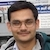# Linear MomentumBy

The linear momentum of a particle of mass $m$ moving with a velocity $\vec{v}$ is given by \begin{align} \vec{p}=m\vec{v}. \end{align}

It is zero for an object at rest. The momentum is low if either mass or velocity (or both) are low. SI unit of linear momentum is kg m/s. Its dimensions are MLT-1. The linear momentum and impulse have the same dimensions.

## Demonstration

This demo gives a feeling of linear momentum.

Take two identical plastic balls. Make a hole in one of them and fill it with sand. Seal the hole with tape. Now you have two balls of different masses. Make a pile of sand on the floor and drop the lighter ball on it from a height. The ball will penetrate into the sand to some depth. Make the pile again and drop the heavier ball on it from the same height. This ball will penetrate deeper into the sand. Dropping from the same height ensures that balls strike the sand with the same velocity. So, increasing the mass increases the depth of penetration when the velocity remains the same.

Now drop one of the balls from different heights on the pile. Dropping from a larger height means the ball strikes the pile with larger velocity. So, increasing the velocity increases the depth of penetration when the mass remains the same.

If you drop the lighter ball from a greater height and the heavier ball from a lower height, it may happen that the depths of penetration become the same. This will happen when the product $$mv$$ is the same.

## Problems from IIT JEE

Problem (JEE 2008): A body of mass $m=3.513$ kg is moving along the $x$-axis with a speed of 5.00 m/s. The magnitude of its linear momentum is recorded as

1. 17.6 kg m/s
2. 17.565 kg m/s
3. 17.56 kg m/s
4. 17.57 kg m/s

Solution: The linear momentum is the product of mass and velocity \begin{align} p&=mv \\ &=(3.513)(5.00)\\ &=17.565 \;\mathrm{kg\,m/s}. \end{align} The number of significant digits in $m=3.513$ kg is four and that in $v=5.00$ m/s is three. As per rules, the product should be truncated to three significant digits (minimum of three and four) i.e., it should be recorded as 17.6 kg m/s.

Problem: An object of mass $m$ moving with speed $v$ strikes a wall at an angle $\theta$ and rebounds at the same speed and same angle. The magnitude of the change in momentum of the object will be

1. $2mv\cos\theta$
2. $2mv\sin\theta$
3. 0
4. $2mv$

Solution: Let $x$ and $y$ axes be as shown in the figure.

The linear momentum of the object before striking the wall is \begin{align} \vec{p}_i=mv\sin\theta\,\hat\imath-mv\cos\theta\,\hat\jmath.\nonumber \end{align} The linear momentum after striking the wall is \begin{align} \vec{p}_f=mv\sin\theta\,\hat\imath+mv\cos\theta\,\hat\jmath.\nonumber \end{align} Thus, the change in linear momentum of the object is \begin{align} \Delta \vec{p}&=\vec{p}_i-\vec{p}_f \\ &=-2mv\cos\theta\,\hat\jmath \end{align} and the magnitude of change in momentum is \begin{align} |\Delta\vec{p}|=2mv\cos\theta. \end{align}

## Related

Subscribe to our channel